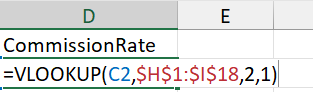# Lesson 12

Preview

VLOOKUP and HLOOKUP Functions

VLOOKUP and HLOOKUP are among the most popular functions used in Excel. These functions enable the user to conveniently associate a piece of data with a category or another important value automatically. VLOOKUP tables are shown in columns and HLOOKUP tables are shown in rows.

• The VLOOKUP is used considerably more than HLOOKUP since the VLOOKUP table is in columns and is easier for users to integrate into their calculations, and it usually takes up much less space than its HLOOKUP counterpart. Because of this fact, we will spend our time with VLOOKUP. The same principles of operations for VLOOKUP also apply to HLOOKUP.
• The VLOOKUP function looks up the value in the first column of the lookup table and returns the corresponding value in a specified table column. The lookup table is arranged vertically (which explains the V in the function’s name). The syntax for the VLOOKUP function is shown on the next page.
• How to interpret what the looked-up value is can confuse some first-time VLOOKUP users if they don’t understand how the logic works. VLOOKUP logic is similar to a Federal Tax Return Tax Table. The looked-up value must be above a certain threshold number, rather than being closer numerically to a number.
• Special Note: If the VLOOKUP 4th argument [range_lookup] is TRUE, perform a sort on your data in the leftmost column from smallest to largest values. If this is not done prior to the VLOOKUP execution, you can have errors in the results. This sort requirement does not apply if the 4th argument is FALSE, or 0.

VLOOKUP Function Argument Explanation (See FIGURE 1 below)

VLOOKUP (lookup_value, table_array, col_index_num, range_lookup (optional) )

lookup_value    (required)

​The value you want to look up. The value you want to look up must be in the first column of the range of cells you specify in table-array . For example, if table-array spans cells B2:D7, then your lookup_value must be in column B. See the graphic above. Lookup_value can be a value or a reference to a cell.
​The range of cells in which the VLOOKUP will search for the lookup_value and the return value.

table_array    (required)

​The first column in the cell range must contain the lookup_value (for example, Last Name in the picture above.) The cell range also needs to include the return value (for example, First Name in the graphic above) you want to find.

col_index_num    (required)

The column number (starting with 1 for the left-most column of table-array) that contains the return value.
A logical value that specifies whether you want VLOOKUP to find an exact match or an approximate match:

range_lookup   (optional)

• TRUE assumes the first column in the table is sorted either numerically or alphabetically, and will then search for the closest value. This is the default method if you don’t specify one.
• FALSE searches for the exact value in the first column.

Practice Exercise 10: Use a VLOOKUP table for applying approximate matching data

Continue to use data file FUNCTIONS.xlsx and select tab VLOOKUP#1 APPROX MATCH.

STEP 1: ​Practice Exercise 10: Use a VLOOKUP table in FIGURE 2 for applying approximate matching data. The VLOOKUP table is in cell addresses H1:I18. The HLOOKUP table is in cell addresses K1: AB2

STEP 2A: (FIGURE 3) Select cell address D2, enter: =VLOOKUP(
STEP 2B: For the 1st argument, lookup_value, we can enter the data value itself or the cell address. In this case, we enter the cell address of the value, by clicking on cell address C2 with the mouse (FIGURE 3).

STEP 2C: Cell address C2 appears as 1st argument (FIGURE 3).

STEP 3A: Enter a comma so it is placed after “C2” (FIGURE 4).

STEP 3B: For the 2nd argument, table_array, enter the range of the VLOOKUP table, H1:I18 (top graphic). We can enter the range by clicking on cell address H1 with the mouse and dragging mouse to cell address I18 (FIGURE 4).

STEP 3C: Highlight range. Press F4 key. Dollar sign symbols should appear for the column and row addresses; \$H\$1:\$I\$18 (FIGURE 4).

STEP 4A: Enter a comma.

STEP 4B: For the 3rd argument, col_index_num, we will enter 2, since our lookup values are in the 2nd column of the lookup table (the left-most column of a lookup table is always column 1) (FIGURE 5).

STEP 5A: Enter a comma.

STEP 5B: For the 4th argument, [range_lookup], understand that this is an optional argument. Since we are doing an approximate match, enter 1 or TRUE, followed by a closed parenthesis. If it was an exact match we’re using, enter a 0 or FALSE.
Note: The default is 1 or TRUE if we do not enter this 4th argument.  Sort data first ​(FIGURE 6).Figure 6 ​CONSIDER IF YOU ARE DOING AN APPROXIMATE MATCH OR EXACT MATCH BEFORE DECIDING WHETHER TO LEAVE THE 4TH ARGUMENT OUT.

STEP 6A: We are ready to execute. Press Enter key. The result in cell address D2 should be .01  (FIGURE 7).

STEP 6B: To change it to a percent, go up to the Number section of the Ribbon, and select the % icon. You should now see 1% in cell address D2 (FIGURE 7).